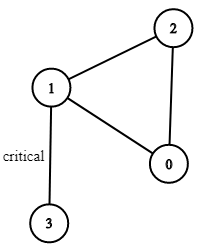# 1192. Critical Connections in a Network

There are `n` servers numbered from `0` to `n - 1` connected by undirected server-to-server `connections` forming a network where `connections[i] = [ai, bi]` represents a connection between servers `ai` and `bi`. Any server can reach other servers directly or indirectly through the network.

critical connection  is a connection that, if removed, will make some servers unable to reach some other server.

Return all critical connections in the network in any order.

Example 1:``````Input: n = 4, connections = [[0,1],[1,2],[2,0],[1,3]]
Output: [[1,3]]
Explanation: [[3,1]] is also accepted.
``````

Example 2:

``````Input: n = 2, connections = [[0,1]]
Output: [[0,1]]
``````

Constraints:

• `2 <= n <= 10^5`
• `n - 1 <= connections.length <= 10^5`
• `0 <= ai, bi <= n - 1`
• `ai != bi`
• There are no repeated connections.

``````class Solution {
public:
vector<vector<int>> criticalConnections(int n, vector<vector<int>>& connections) {
int cnt = 1;
vector<vector<int>> res;
vector<int> time(n), low(n);
unordered_map<int, vector<int>> g;
for (auto conn : connections) {
g[conn].push_back(conn);
g[conn].push_back(conn);
}
helper(g, 0, -1, cnt, time, low, res);
return res;
}
void helper(unordered_map<int, vector<int>>& g, int cur, int pre, int& cnt, vector<int>& time, vector<int>& low, vector<vector<int>>& res) {
time[cur] = low[cur] = cnt++;
for (int next : g[cur]) {
if (time[next] == 0) {
helper(g, next, cur, cnt, time, low, res);
low[cur] = min(low[cur], low[next]);
} else if (next != pre) {
low[cur] = min(low[cur], time[next]);
}
if (low[next] > time[cur]) {
res.push_back({cur, next});
}
}
}
};
``````

Github 同步地址:

https://github.com/grandyang/leetcode/issues/1192

https://leetcode.com/problems/critical-connections-in-a-network/

https://zhuanlan.zhihu.com/p/101923309

https://leetcode.com/problems/critical-connections-in-a-network/discuss/382638/DFS-detailed-explanation-O(orEor)-solution

https://leetcode.com/problems/critical-connections-in-a-network/discuss/1174196/JS-Python-Java-C%2B%2B-or-Tarjan's-Algorithm-Solution-w-Explanation

LeetCode All in One 题目讲解汇总(持续更新中…)

 微信打赏Venmo 打赏×

Help us with donation